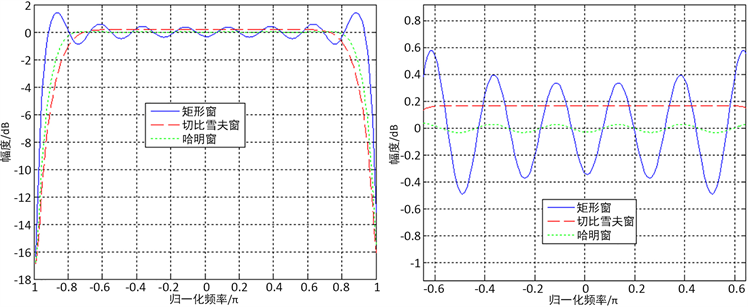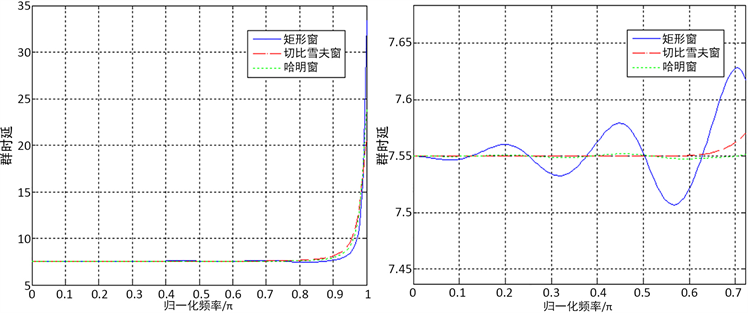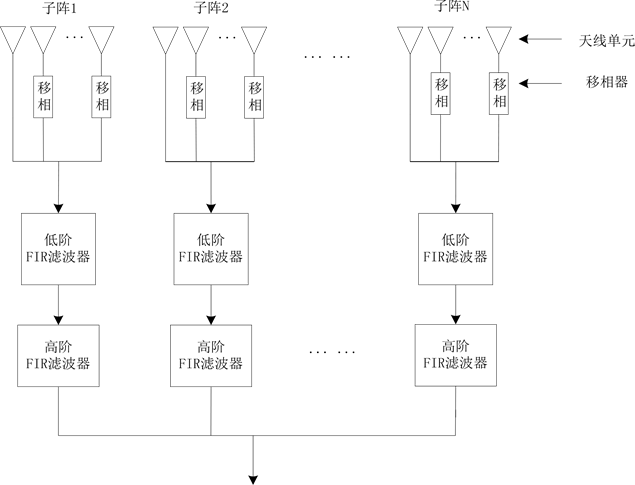﻿ 一种全数字滤波器的共形阵宽带阵列波束合成方法

# 一种全数字滤波器的共形阵宽带阵列波束合成方法A Wideband Digital Array Beamforming Method of Conformal Array Antenna Based on Digital Filters

Abstract: In wideband signal beamforming, the traditional phase-shift method causes pattern pointing dev-iation and sidelobe widening, so the true time delay should be used to compensate the cannel delay accurately. In connection with low computation and high time delay precision of the wideband digital array beamforming, this paper proposed a digital beamforming system with high-order and low-order filter cascades. The simulation result indicates that the method proposed in this paper can solve effectively the pattern pointing deviation and sidelobe widening problems.

1. 引言

2. 时延滤波器原理

$h\left(n\right)=\text{sinc}\left(n-Dela{y}_{i}\right)$ (1)

$Dela{y}_{i}$ 不是整数时， $h\left(n\right)$ 是非因果的，实际中，通常用加窗来实现(窗函数可以选矩形窗、汉明窗、切比雪夫窗等)。即分数时延滤波器的冲激响应可以表示为

$h\left(n\right)=Window\left(n\right)×\text{sinc}\left(n-Dela{y}_{i}\right)$ (2)

$h\left(n\right)=Window\left(n\right)×\text{sinc}\left(n-dela{y}_{i}-L\right),\text{ }\text{ }\text{ }\text{ }\text{ }\text{ }\text{ }\text{ }\text{ }\text{ }\text{ }\text{ }n=0,1,\cdots ,2L$ (3)(a) (b)

Figure 1. (a) Amplitude frequency response; (b) Partial enlarged view of amplitude frequency response(a) (b)

Figure 2. (a) Group delay response; (b) Partial enlarged view of group delay response

3. 系统原理

3.1. 子阵划分原理

$\Delta f\le \frac{c}{L\cdot \mathrm{sin}{\theta }_{B}}$ (4)

3.2. 低阶滤波器实现数字延迟线原理Figure 3. Wideband beamforming method of conformal array antenna

${N}_{\mathrm{max}}=\frac{5×\mathrm{sin}{75}^{\circ }}{3×{10}^{8}}×200×{10}^{6}=\text{3}\text{.2198}$ (5)

$h\left(n\right)=\mathrm{sinc}\left(n-N\right),\text{ }\text{ }\text{ }\text{ }\text{ }\text{ }\text{ }\text{ }\text{ }\text{ }n=0,1,\cdots ,\text{round}\left({N}_{\mathrm{max}}\right)$ (6)

3.3. 高阶滤波器实现分数延迟原理

$h\left(n\right)=Window\left(n\right)×\text{sinc}\left(n-Dela{y}_{i}-M\right),\text{ }\text{ }\text{ }\text{ }\text{ }\text{ }\text{ }\text{ }\text{ }n=0,1,\cdots ,2×M$ (7)

4. 工作流程

5. 系统仿真与验证Figure 4. Array pattern contrast

6. 结束语

 唐波, 汤俊, 唐猛, 彭应宁. 共形相控阵信号处理技术研究[J]. 航空科学技术, 2009(5): 36-40.

 Quazi, A.H. (1981) An Overview on the Time Delay Estimate in Active and Passive Systems for Target Localization. IEEE Transactions on Acoustics, Speech, and Signal Processing, 29, 527-533.
https://doi.org/10.1109/TASSP.1981.1163618

 曹运合, 李强, 王胜华, 等. 宽带相控阵雷达发射波束零点形成方法[J]. 西安电子科技大学学报: 自然科学版, 2006, 33(3): 395-399.

 董汉清. 跳扩频测控信号中伪码同步技术的研究与仿真[D]: [硕士学位论文]. 成都: 电子科技大学, 2013: 1.

 刘张林. 基于分数时延的宽带数字波束形成技术[J]. 现代电子技术, 2013(3): 24-26.

Top